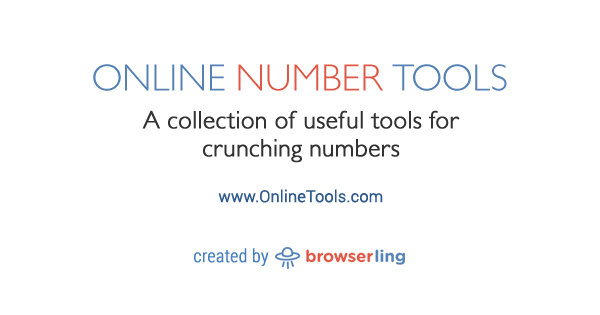At Browserling we’re building a network of online tools websites. Each in the network focuses on one and only one tool category. Each tool does one and only one thing. The first sixteen websites in the network are Online CSV Tools, which is all about working with Comma Separated Values files, Online TSV Tools, which is all about working with Tab Separated Values files, Online JSON Tools, which is all about working with JSON , Online XML Tools, which is all about working with XML documents, Online YAML Tools, which is all about working with YAML configs, Online STRING Tools, which is all about working with strings, Online RANDOM Tools, which is all about doing random things, Online BINARY Tools, which is all about working with binary zeros and ones, Online PNG Tools, which is all about working with PNG images, Online HEX Tools, which is all about working with hexadecimal values, Online JPG Tools, which is all about working with JPEG images, Online ASCII Tools, which is all about working with ASCII charset, Online MATH Tools, which is all about doing mathy things, Online IMAGE Tools, which is all about working with various image formats, Online UTF8 Tools, which is all about working with UTF8 encoding, Online TEXT Tools, which is all about doing text processing.

Today we’re releasing the seventeenth site.

The seventeenth site in our network is Online Number Tools. Number is a collection of simple, free and easy to use utilities for doing all kinds of numbery things. There are no ads, popups or other garbage. Just number utilities that work in your browser. Load numbers and instantly get result!www.onlineNUMBERtools.com

Here’s a list of all number tools so far:

Here are upcoming number tools:

• Check if a Number is Numberwang
• Shuffle Digits in a Number
• Rewrite Numbers
• Generate Powers of 2
• Generate Powers of
• Create a Floating Point Number
• Convert a Number to the Scientific Notation
• Convert Scientific Notation to a Number
• Generate Random Binary Numbers
• Generate Random Octal Numbers
• Generate Random Decimal Numbers
• Generate Random Hex Numbers
• Calculate a Running Sum
• Calculate a Running Product
• Find the Maximal Number
• Find the Minimal Number
• Calculate the Factorial
• Generate Number Bigrams
• Generate Number Trigrams
• Generate Number N-grams
• Analyze a Number
• Convert a Number to an Ordinal
• Convert an Ordinal to a Number
• Convert a Number to Words
• Convert Words to a Number
• Convert a Number to Roman Number
• Convert a Roman Number to Regular Number
• Generate Negafibonacci Numbers
• Generate Fibonacci Primes
• Fibonacci Number Test
• Fibonacci Prime Test
• Construct Fibonacci Words
• Construct Tribonacci Words
• Generate Negalucas Numbers
• Generate Lucas Primes
• Lucas Prime Test
• Invert a Matrix
• Calculate the Trace of a Matrix
• Calculate the Eigenvalues of a Matrix
• Multiply Matrices
• Add Matrices
• Subtract Matrices
• Generate Moser de Bruijn Numbers
• Generate Kolakoski Numbers
• Generate Gijswijt Numbers
• Generate Rudin-Shapiro Numbers
• Generate Baum-Sweet Numbers
• Generate Thue-Morse Sequence
• Generate Perfect Numbers
• Generate Almost Perfect Numbers
• Generate Excessive Number Sequence
• Generate Deficient Number Sequence
• Calculate Dragon Curve Numbers
• Generate Composite Numbers
• Test if a Number is Perfect
• Test if a Number is Abundant
• Test if a Number is Deficient
• Calculate Greatest Common Divisor
• Calculate Least Common Multiple
• Generate a Sequence of Squares
• Generate a Sequence of Cubes
• Calculate the Modulo
• Group Number Digits
• Split a Number into Digits
• Printf Numbers
• Palindrome Number Test
• Create a Palindrome Number
• Create Zalgo Numbers
• Mirror a Number
• Reverse a Number
• Rotate a Number
• Increase All Digits in a Number
• Decrease All Digits in a Number
• Calculate Percentages
• Generate Natural Numbers
• Generate Rational Numbers
• Generate Numbers
• Generate Complex Numbers
• Generate Binary Numbers
• Generate Pairs of Numbers
• Convert a Fraction to a Decimal
• Convert a Decimal to a Fraction
• Convert a Binary Number to Octal Number
• Convert a Binary Number to Decimal Number
• Convert a Binary Number to Hex Number
• Convert a Octal Number to Binary Number
• Convert a Octal Number to Decimal Number
• Convert a Octal Number to Hex Number
• Convert a Decimal Number to Binary Number
• Convert a Decimal Number to Octal Number
• Convert a Decimal Number to Hex Number
• Convert a Hex Number to Binary Number
• Convert a Hex Number to Octal Number
• Convert a Hex Number to Decimal Number
• Convert Any Number to Any Base
• Number’s Mantissa
• Change Number’s Base
• Change Number’s Exponent
• Generate Numberwang Numbers

The next few sites are onlineHASHtools, onlineGIFtools, onlineBMPtools, onlinePDFtools, onlineBROWSERtools, onlineCRYPTOtools, onlineAUDIOtools, onlineCSStools, onlineJStools, and then 999 more.

See you next !

SHARE